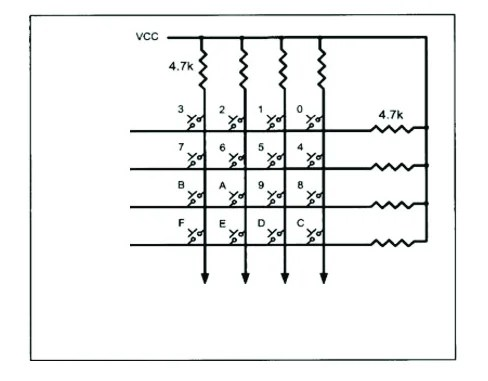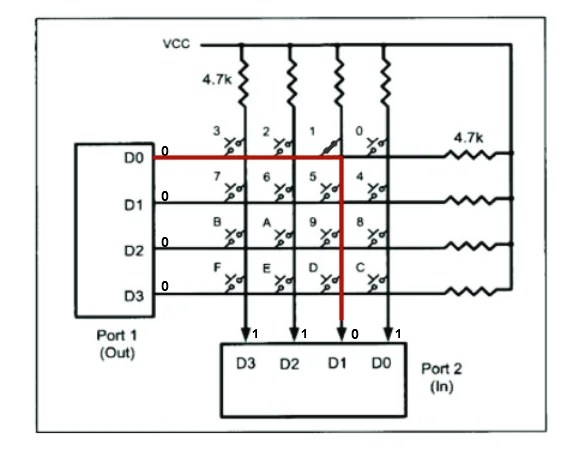View Course Path

# Interfacing 4×4 Keypad matrix with 8051 microcontroller

When it comes to input/output devices, there’s a plethora of them available in the market. From DC motors to LCDs, I/O devices enhance the functionality of any microcontroller. In this article, we will learn everything about interfacing a 4×4 matrix keypad (hex-keypad) with the 8051 microcontroller. Before we get into the whole interfacing mumbo jumbo, let us look at the basics of how a keypad works and how the microcontroller extracts information from it.

Contents

## What is a Keypad, and how does it work?

If you look at it, a keypad is nothing but a bunch of switches arranged in a particular fashion. So you might be wondering why use this pattern? Why not connect the switches directly to the ports and detect when a key is pressed. I mean it would be easy right. Well if you were to do that, you would need 16 ports to monitor 16 switches, and if you remember from earlier in this 8051 course, we only have 32 ports on our microcontroller. Therefore it would be better to arrange these switches in a more optimized pattern so that we need a lesser number of pins to monitor these switches.If you look at the image given above, you can see a bunch of switches arranged in a 4×4 matrix. Pressing a switch on the keypad creates a short circuit which is detected by the microcontroller. Now that we have the basics of how the keys are arranged in a matrix, let us look at how the microcontroller detects a keypress.

## The logic behind detecting a keypress using a microcontroller

To detect the key that’s pressed, two ports of the microcontroller are connected to the rows and columns of the matrix respectively. The port which is connected to the rows of the matrix is configured as an output port, hence making each row logic 0. On the contrary, the port which is connected to the columns is configured as an input port, making the column at logic 1.When a button is pressed, it changes the logic of that particular column to 0 because the button causes a short circuit between the row and the column. As an example, let us say the key with the number 1 is pressed. This causes the D1 column to go to logic 0 as it shorts row D0 with it.For Port 2 the values are currently D3D2D1D0 = 1101. The 0 indicates that a button in the D1 column is pressed. So we have identified the column now. Next, we will identify the row.

Looking at the picture above, you might be wondering that the same output would be produced if any of the keys in the column D1 were pressed. To find the row which has the pressed key, the microcontroller grounds the rows one by one and checks in which case the column is logic zero. This helps the microcontroller to find which key was pressed.

To summarize, when a key is pressed, the column containing the key is driven to logic 0. The microcontroller then checks each row and identifies the row where the button is pressed.

Now that we have the basic logic behind detecting a keypress, let us look at an algorithm that can be used to detect keypresses in a robust manner.

## Algorithm to detect a keypress using a keypad

Although we have understood the basic idea behind detecting a keypress using a matrix keypad, doing it in that manner poses a few other problems which our algorithm needs to solve for proper detection.

• Debouncing: The main job of any switch is to connect two terminals to complete a circuit. But here is the issue, this process is not instantaneous. What this means is that it takes some time for the switch to complete the circuit. Due to this, the signal to the microcontroller is noisy until the contacts of the switch make proper contact with the underlying wires. Although this problem can be solved using capacitors and Schmitt triggers, it is better to use a software approach to solve the problem. To solve this problem, the microcontroller needs to wait for a certain amount of time and allow the signal to stabilize.
• Making sure the previous key has been released: The microcontroller needs to start scanning the matrix after a keypress has been detected. To do this, the microcontroller must make all the rows logic zero and see if all the columns have a logic 1. If all the columns have logic 1, it means that none of the keys are pressed, and the microcontroller needs to look for a new keypress.
• Encoding the keypress: detecting a keypress is one thing, but it is also essential to convert the information about the keypress into information that can be shown on an LCD or any other output peripheral for that matter. Here’s a flowchart that will help you understand the entire process of detecting a keypress. It’s simpler than it looks.Now that we have all that we need let us get on with the interfacing

## Interfacing 8051 with 4×4 matrix keypadTo interface a  4×4 keypad to 8051, follow these steps. In our case, we are using Proteus as the simulation software and the AT89C51 microcontroller.

• Connect the oscillator circuit to pins 19 and 20. This includes a crystal oscillator and two capacitors of 22uF each. Connect them to the pins, as shown in the diagram.
• Connect one end of the capacitor to the EA’ pin and the other to the resister. Connect this resistor to the RST pin, as shown in the diagram.
• Connect the column matrix to the lower nibble of port 3
• Connect the row matrix to the lower nibble of port 2

## Interfacing 8051 with 4×4 matrix keypad and LCDConnect the LCD to port 1 of the microcontroller. To get a better understanding of the interfacing of an LCD, go through our article on interfacing 8051 with LCD. Here’s the C code to display data on an LCD from a 4×4 keypad matrix.

```#include <reg51.h>

void MSDelay(unsigned int );
void LCDtransfer(unsigned char );
void send_command(unsigned char);
void send_data(unsigned char);
sfr COL = 0xB0;
sfr ROW = 0xA0;
sfr ldata = 0x90; //P1 = LCD dat pins
sbit rs = P0^2;
sbit rw = P0^1;
sbit en = P0^0;
'4','5','6','x',
'1','2','3','-',
'e','0','=','+'};
void main()
{
unsigned char collocation,rowlocation;
COL = 0xFF;// Sents port 3 as output port
while(1)
{
do
{
ROW =0X00; //sets the row matrix as an input port
collocation = COL;
collocation &= 0x0F;
}while(collocation != 0x0F);// waits for one of the coloumns to be logic 0
do
{
do
{
MSDelay(20);
collocation = COL;
collocation &= 0x0F;
}while(collocation == 0x0F);
MSDelay(20); // delay fucntion to prevent debouncing
collocation = COL;
collocation &=0x0F;
}while(collocation == 0x0F);
while(1) // finds which row the key belong to
{
ROW = 0XFE;
collocation = COL;
collocation &=0x0F;
if(collocation != 0x0F)
{
rowlocation = 0;
break;
}
ROW = 0XFD;
collocation = COL;
collocation &=0x0F;
if(collocation != 0x0F)
{
rowlocation = 1;
break;
}
ROW = 0XFB;
collocation = COL;
collocation &=0x0F;
if(collocation != 0x0F)
{
rowlocation = 2;
break;
}
ROW = 0XF7;
collocation = COL;
collocation &=0x0F;
rowlocation = 3;
break;
}
send_command(0x38); // 16_bit mode; Enable 5x7 dot matrix for each character
MSDelay(250);
send_command(0x0E); // Display ON & cursor ON
MSDelay(250);
send_command(0x01); // Clear the Display
MSDelay(250);
send_command(0x06); // To shift the cursor right
MSDelay(250);
send_command(0x83); // Set the cursor at line 1, position 3
MSDelay(250);
if(collocation ==0x0E)
else if(collocation == 0x0D)
else if(collocation == 0x0B)
else
}
}

void MSDelay(unsigned int value)
{
unsigned int x, y;
for(x=0;x<1275;x++)
for(y=0;y<value;y++);

}
void LCDtransfer(unsigned char keypress)
{
ldata = keypress;
rs = 1;
rw = 0;
en = 1;
MSDelay(10);
en = 0;
return;
}
void send_command(unsigned char value)
{
ldata = value;
rs = 0;
rw = 0;
en = 1;
MSDelay(10);
en = 0;
return;
}```